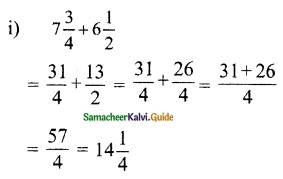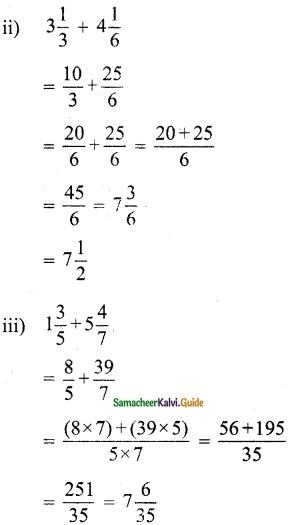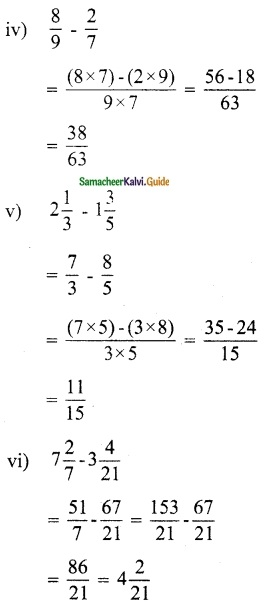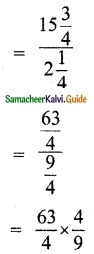Students can download Maths Chapter 1 Fractions Ex 1.1 Questions and Answers, Notes, Samacheer Kalvi 6th Maths Guide Pdf helps you to revise the complete Tamilnadu State Board New Syllabus, helps students complete homework assignments and to score high marks in board exams.

## Tamilnadu Samacheer Kalvi 6th Maths Solutions Term 3 Chapter 1 Fractions Ex 1.1

Question 1.
Fill in the blanks:
(i) 7$$\frac{3}{4}$$ + 6$$\frac{1}{2}$$ ………..
(ii) The sum of a whole number and a proper fraction is called ……….
(iii) 5$$\frac{1}{3}$$ – 3$$\frac{1}{2}$$ ………..
(iv) 8 ÷ $$\frac{1}{2}$$ ………..
(v) The number which has its own reciprocal is ……….
Solution:(ii) Mixed Fraction

(iii) 5$$\frac{1}{3}$$ – 3$$\frac{1}{2}$$
= $$\frac{16}{3}$$ – $$\frac{7}{2}$$ = $$\frac{32-21}{6}$$ = $$\frac{11}{6}$$
= 1$$\frac{5}{6}$$

(iv) 8 ÷ $$\frac{1}{2}$$
= 8 × $$\frac{2}{1}$$
= 16

(v) 1The first step would be to write the mix number 223 as an improper fraction.

Question 2.
Say True or False
(i) 3$$\frac{1}{2}$$ can be written as 3 + $$\frac{1}{2}$$.
(ii) The sum of any two proper fractions is always an improper fraction.
(iii) The mixed fraction of $$\frac{13}{4}$$ is 3$$\frac{1}{4}$$.
(iv) The reciprocal of an improper fraction is always a proper fraction.
(v) 3$$\frac{1}{4}$$ × 3$$\frac{1}{4}$$ = 9$$\frac{1}{16}$$
Solution:
(i) True
(ii) False
(iii) True
(iv) True
(v) False

Question 3.
(i) Find the sum of $$\frac{1}{7}$$ and $$\frac{3}{9}$$.
(ii) What is the total of 3$$\frac{1}{3}$$ and 4$$\frac{1}{6}$$?
(iii) Simplify: 1$$\frac{3}{5}$$+5$$\frac{4}{7}$$.
(iv) Find the difference between $$\frac{8}{9}$$ and $$\frac{2}{7}$$
(v) Subtract: 1$$\frac{3}{5}$$ from 2$$\frac{1}{3}$$.
(vi) Simplify: 7$$\frac{2}{7}$$ – 3$$\frac{4}{21}$$
Solution:
(i) $$\frac{1}{7}$$ + $$\frac{3}{9}$$
= $$\frac{9+21}{63}$$ = $$\frac{30}{63}$$ = $$\frac{10}{21}$$Question 4.
Convert mixed fractions into improper fractions and vice versa:
(i) 3$$\frac{7}{18}$$
(ii) $$\frac{99}{7}$$
(iii) $$\frac{47}{6}$$
(iv) 12$$\frac{1}{9}$$
Solution:
(i) 3$$\frac{7}{18}$$ = $$\frac{61}{18}$$
(ii) $$\frac{99}{7}$$ = 14$$\frac{1}{7}$$
(iii) $$\frac{47}{6}$$ = 7$$\frac{5}{6}$$
(iv) 12$$\frac{1}{9}$$ = $$\frac{109}{9}$$

Question 5.
Multiply the following:
(i) $$\frac{2}{3}$$ × 6
(ii) 8$$\frac{1}{3}$$ × 5
(iii) $$\frac{3}{8}$$ × $$\frac{4}{5}$$
(iv) 3$$\frac{5}{7}$$ × 1$$\frac{1}{13}$$
Solution:
(i) $$\frac{2}{3}$$ × 6 = 4

(ii) 8$$\frac{1}{3}$$ × 5
= $$\frac{25}{3}$$ × 5
= $$\frac{125}{3}$$
= 41$$\frac{2}{3}$$

(iii) $$\frac{3}{8}$$ × $$\frac{4}{5}$$
= $$\frac{12}{40}$$ = $$\frac{3}{10}$$

(iv) 3$$\frac{5}{7}$$ × 1$$\frac{1}{13}$$
= $$\frac{26}{7}$$ × $$\frac{14}{13}$$
= $$\frac{4}{1}$$
= 4Question 6.
Divide the following
(i) $$\frac{3}{7}$$ ÷ 4
(ii) $$\frac{4}{3}$$ ÷ $$\frac{5}{9}$$
(iii) 4$$\frac{1}{5}$$ ÷ 3$$\frac{3}{4}$$
(iv) 9$$\frac{2}{3}$$ ÷ 1$$\frac{2}{3}$$
Solution:
(i) $$\frac{3}{7}$$ ÷ 4
= $$\frac{3}{7}$$ × $$\frac{1}{4}$$ = $$\frac{3}{28}$$

(ii) $$\frac{4}{3}$$ ÷ $$\frac{5}{9}$$
= $$\frac{4}{3}$$ × $$\frac{9}{5}$$
= $$\frac{12}{5}$$
= 2$$\frac{2}{5}$$Question 7.
Gowri purchased 3$$\frac{1}{2}$$ kg of tomatoes, $$\frac{3}{4}$$ kg of brinjal and 1$$\frac{1}{4}$$ kg of onion. What is the total weight of the vegetables she bought?
Solution:
Total weight of vegetables boughtQuestion 8.
An oil tin contains 3$$\frac{3}{4}$$ litres of oil of which 2$$\frac{1}{2}$$ litres of oil is used. How much oil is left over?
Solution:Question 9.
Nilavan can walk 4$$\frac{1}{2}$$ km in an hour. How much distance will he cover in 3$$\frac{1}{2}$$ hours?
Solution:
Distance walked in an hour = 4$$\frac{1}{2}$$ km
Distance covered in 3$$\frac{1}{2}$$
HoursQuestion 10.
Ravi bought a curtain of length 15$$\frac{3}{4}$$ m. If he cut the curtain into small pieces each of length 2$$\frac{1}{4}$$ m, then how many small curtains will he get?
Solution:
Total length = 15$$\frac{3}{4}$$
Length of the small pieces = 2$$\frac{1}{4}$$
Small curtains obtained= 7Objective Type Questions

Question 11.
Which of the following statement is incorrect?
(a) $$\frac{1}{2}$$ > $$\frac{1}{3}$$
(b) $$\frac{7}{8}$$ > $$\frac{6}{7}$$
(c) $$\frac{8}{9}$$ < $$\frac{9}{10}$$
(d) $$\frac{10}{11}$$ > $$\frac{9}{10}$$
Solution:
(d) $$\frac{10}{11}$$ > $$\frac{9}{10}$$

Question 12.
The difference between $$\frac{3}{7}$$ and $$\frac{2}{9}$$ is
(a) $$\frac{13}{63}$$
(b) $$\frac{1}{9}$$
(c) $$\frac{1}{7}$$
(d) $$\frac{9}{16}$$
Solution:
(a) $$\frac{13}{63}$$Question 13.
The reciprocal of $$\frac{53}{17}$$ is
(a) $$\frac{53}{17}$$
(b) 5$$\frac{3}{17}$$
(c) $$\frac{17}{53}$$
(d) 3$$\frac{5}{17}$$
Solution:
(c) $$\frac{17}{53}$$

Question 14.
If $$\frac{6}{7}=\frac{\mathbf{A}}{49}$$, then the value of A is
(a) 42
(b) 36
(c) 25
(d) 48
Solution:
(a) 42
Hint: $$\frac{6 \times 49}{7}=42$$Question 15.
Pugazh has been given four choices for his pocket money by his father. Which of the choices should he take in order to get the maximum money?
(a) $$\frac{2}{3}$$ of Rs 150
(b) $$\frac{3}{5}$$ of Rs 150
(c) $$\frac{4}{5}$$ of Rs 150
(d) $$\frac{1}{5}$$ of Rs 150
Solution:
(c) $$\frac{4}{5}$$ of Rs 150